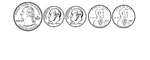### 47 Cents

Groups of change with totals from 1 to 100 cents using the least amount of coins.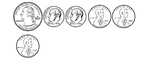### 48 Cents

Groups of change with totals from 1 to 100 cents using the least amount of coins.### 49 Cents

Groups of change with totals from 1 to 100 cents using the least amount of coins.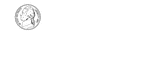### 5 Cents

Groups of change with totals from 1 to 100 cents using the least amount of coins.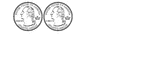### 50 Cents

Groups of change with totals from 1 to 100 cents using the least amount of coins.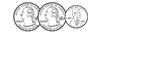### 51 Cents

Groups of change with totals from 1 to 100 cents using the least amount of coins.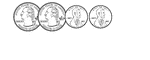### 52 Cents

Groups of change with totals from 1 to 100 cents using the least amount of coins.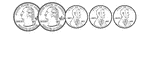### 53 Cents

Groups of change with totals from 1 to 100 cents using the least amount of coins.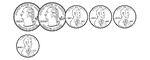### 54 Cents

Groups of change with totals from 1 to 100 cents using the least amount of coins.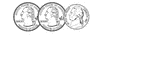### 55 Cents

Groups of change with totals from 1 to 100 cents using the least amount of coins.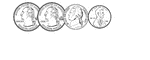### 56 Cents

Groups of change with totals from 1 to 100 cents using the least amount of coins.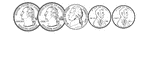### 57 Cents

Groups of change with totals from 1 to 100 cents using the least amount of coins.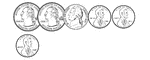### 58 Cents

Groups of change with totals from 1 to 100 cents using the least amount of coins.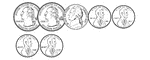### 59 Cents

Groups of change with totals from 1 to 100 cents using the least amount of coins.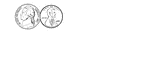### 6 Cents

Groups of change with totals from 1 to 100 cents using the least amount of coins.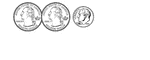### 60 Cents

Groups of change with totals from 1 to 100 cents using the least amount of coins.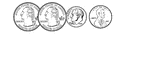### 61 Cents

Groups of change with totals from 1 to 100 cents using the least amount of coins.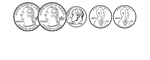### 62 Cents

Groups of change with totals from 1 to 100 cents using the least amount of coins.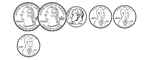### 63 Cents

Groups of change with totals from 1 to 100 cents using the least amount of coins.### 64 Cents

Groups of change with totals from 1 to 100 cents using the least amount of coins.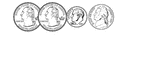### 65 Cents

Groups of change with totals from 1 to 100 cents using the least amount of coins.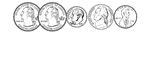### 66 Cents

Groups of change with totals from 1 to 100 cents using the least amount of coins.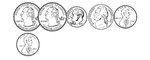### 67 Cents

Groups of change with totals from 1 to 100 cents using the least amount of coins.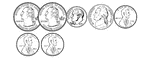### 68 Cents

Groups of change with totals from 1 to 100 cents using the least amount of coins.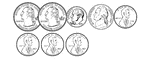### 69 Cents

Groups of change with totals from 1 to 100 cents using the least amount of coins.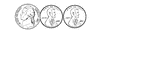### 7 Cents

Groups of change with totals from 1 to 100 cents using the least amount of coins.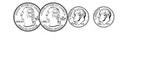### 70 Cents

Groups of change with totals from 1 to 100 cents using the least amount of coins.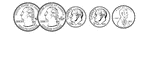### 71 Cents

Groups of change with totals from 1 to 100 cents using the least amount of coins.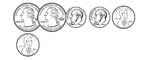### 72 Cents

Groups of change with totals from 1 to 100 cents using the least amount of coins.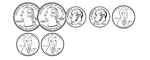### 73 Cents

Groups of change with totals from 1 to 100 cents using the least amount of coins.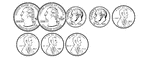### 74 Cents

Groups of change with totals from 1 to 100 cents using the least amount of coins.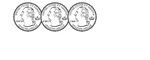### 75 Cents

Groups of change with totals from 1 to 100 cents using the least amount of coins.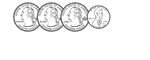### 76 Cents

Groups of change with totals from 1 to 100 cents using the least amount of coins.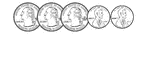### 77 Cents

Groups of change with totals from 1 to 100 cents using the least amount of coins.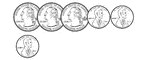### 78 Cents

Groups of change with totals from 1 to 100 cents using the least amount of coins.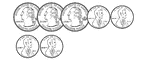### 79 Cents

Groups of change with totals from 1 to 100 cents using the least amount of coins.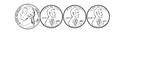### 8 Cents

Groups of change with totals from 1 to 100 cents using the least amount of coins.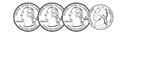### 80 Cents

Groups of change with totals from 1 to 100 cents using the least amount of coins.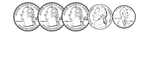### 81 Cents

Groups of change with totals from 1 to 100 cents using the least amount of coins.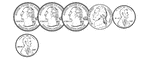### 82 Cents

Groups of change with totals from 1 to 100 cents using the least amount of coins.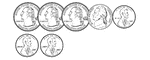### 83 Cents

Groups of change with totals from 1 to 100 cents using the least amount of coins.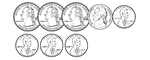### 84 Cents

Groups of change with totals from 1 to 100 cents using the least amount of coins.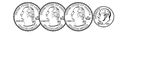### 85 Cents

Groups of change with totals from 1 to 100 cents using the least amount of coins.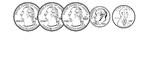### 86 Cents

Groups of change with totals from 1 to 100 cents using the least amount of coins.### 87 Cents

Groups of change with totals from 1 to 100 cents using the least amount of coins.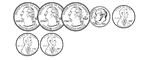### 88 Cents

Groups of change with totals from 1 to 100 cents using the least amount of coins.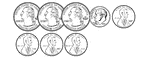### 89 Cents

Groups of change with totals from 1 to 100 cents using the least amount of coins.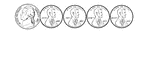### 9 Cents

Groups of change with totals from 1 to 100 cents using the least amount of coins.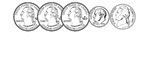### 90 Cents

Groups of change with totals from 1 to 100 cents using the least amount of coins.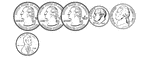### 91 Cents

Groups of change with totals from 1 to 100 cents using the least amount of coins.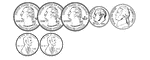### 92 Cents

Groups of change with totals from 1 to 100 cents using the least amount of coins.### 93 Cents

Groups of change with totals from 1 to 100 cents using the least amount of coins.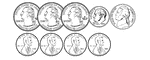### 94 Cents

Groups of change with totals from 1 to 100 cents using the least amount of coins.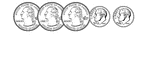### 95 Cents

Groups of change with totals from 1 to 100 cents using the least amount of coins.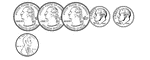### 96 Cents

Groups of change with totals from 1 to 100 cents using the least amount of coins.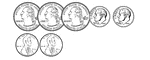### 97 Cents

Groups of change with totals from 1 to 100 cents using the least amount of coins.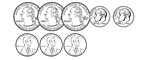### 98 Cents

Groups of change with totals from 1 to 100 cents using the least amount of coins.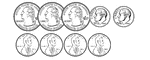### 99 Cents

Groups of change with totals from 1 to 100 cents using the least amount of coins.### Charles II

"Coin of Charles II." — Lardner, 1885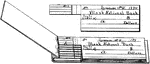### Check Book

A check is a negotiable instrument instructing a financial institution to pay a specific amount of a…